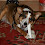## Saturday, May 19, 2007

### Infinity ain't everything

Steve Esser drops an interesting comment at FQI:
If the actual universe is infinite, wouldn’t it contain all possibilities?

Not necessarily. A collection may be infinite whilst making systematic exclusions. Consider the set of even numbers. It's infinite in number, but it doesn't contain all the numbers. Granted, the odd numbers never really had a chance, in this artificial case. So perhaps the thought is instead that an infinite expanse will contain every finite pattern that it possibly could. This seems more intuitive. But in fact this too turns out to be false.

Consider an infinite sequence of coin-flips. Suppose it is a fair coin, so for each individual flipping event, there is a 50/50 chance of it landing heads or tails. So one obvious possibility is for a "tails" event to occur. But is it guaranteed to occur, at some point in the infinite sequence? Well, no. There is, after all, some (infinitesimal) chance that the coin will land heads on every single flip whatsoever! In this case, all the other possibilities that might have obtained, won't. So, infinity is no guarantee of actualizing every possibility.

1.Are you sure you can generalize the coin-flip reasoning to an actual infinity? I don't have a full grasp of the mathematics, but the impression I have is that infinitesimals are only good when you're approaching infinity, not at actual infinity.

So in this case, the probability of at least one coin being tails approaches one as the number of coin flips approaches infinity, retaining the infinitesimal possibility of all the flips being heads. However, for an infinite number of flips, the probability of at least one coin being tails is one, and there is a zero probability of all the flips being heads.

Intuition says that there is always the chance of getting yet another heads, but intuition tends to break down with true infinity.

2.I'm not sure. But note that the "all heads" outcome is not any less likely than any other particular (fully specified) outcome. And some or other particular outcome must result. So it can't be impossible. (It may be "probability zero" in some weird mathematical sense that doesn't entail actual impossibility.)

Here's another example: a random sequence of natural numbers isn't guaranteed to "eventually" contain the number 7. For there's some (again, infinitesimal) chance that the sequence will just happen to mimic the sequence of even numbers. And in that case, wait as long as you like, the number 7 will never, ever, show up.

3.> But is it guaranteed to occur, at some point in the infinite sequence? Well, no.

In your example is it not as or more guaranteed than anything else you have ever guaranteed in your life? I also presume the x/infinity probably isn't large enough to make Steve feel any better about it.

GNZ

4.Ha, yeah, fair point!

5.Hi Richard. I think your first point is right that one can restrict the class of possibilities in the infinite set. In the context of a world, there still may be limits of this sort, I guess. For instance if laws of nature are fixed and not variable. This is certainly a difficult topic.
- Steve Esser

6.One has to ask what one means by an actual universe being infinite. Are we speaking temporally, spatially or something like the many-worlds interpretation of quantum mechanics?

After all if we're just talking about temporal then we'd have very limited possibilities if the universe just reaches heat death. That is still infinite but hardly encompasses all possibilities.

The spatially infinite universe also would seem to limit possibilities. Certainly not everything would happen.

So it seems by "infinite" one is talking in a more Lewis sense. In a Lewis sense what the person says is true but almost no one is talking about Lewis' sort of realism towards possibilities when they talk about infinite. Certainly the MWI is more narrow.

I think Tipler goes in the direction of dealing with finite patterns. But as you note that is false. (I'm not sure what exactly Tipler's position is, I should note)

7.There is a very precise way in which infinite sets can be of “different sizes”. Notably, the set of real numbers is bigger than the set of counting numbers. What this means is that if you try and create a one to one correspondence between counting numbers and real numbers, it is always possible to show that you have missed some real numbers even though your list of counting numbers is infinitely long. Look at Cantor’s diagonal argument” if you want to see how this is done—it is a clever proof.

http://en.wikipedia.org/wiki/Cantor_diagonal_argument

So yes, it is possible to have infinite sets that do not contain all the elements of larger infinite sets. I guess the question then is would infinite space/time be such a set? I know physicists have considered the question, but I don’t know if current theory points to much of an answer.

Visitors: check my comments policy first.
Non-Blogger users: If the comment form isn't working for you, email me your comment and I can post it on your behalf. (If your comment is too long, first try breaking it into two parts.)